## Example Questions

2 Next →

### Example Question #11 : Working With Fractions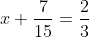For what value of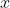is this equation true?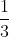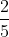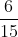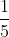Explanation:

To solve this problem we must first set a common denominator. To set a common denominator we multiply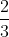by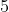, by doing this we get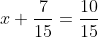and see that the common denominator is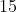. Then, since the denominators are now the same, we subtract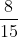from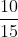and move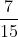to the other side to get thealone and simultaneously solve for.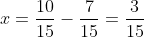We simplify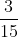by dividing the numerator and denominator by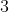, and get the answer.

2 Next →

### All SAT Mathematics Resources